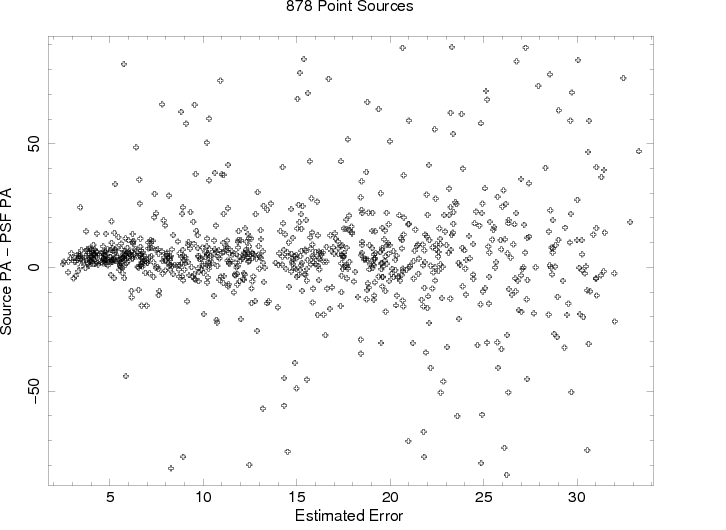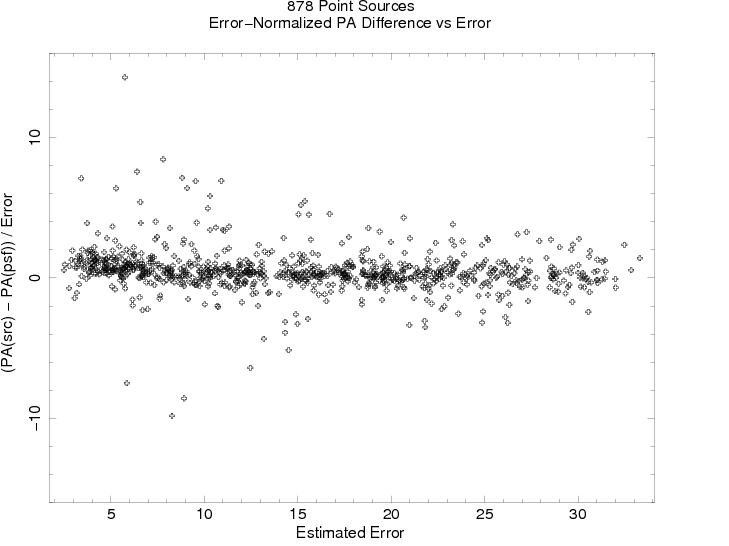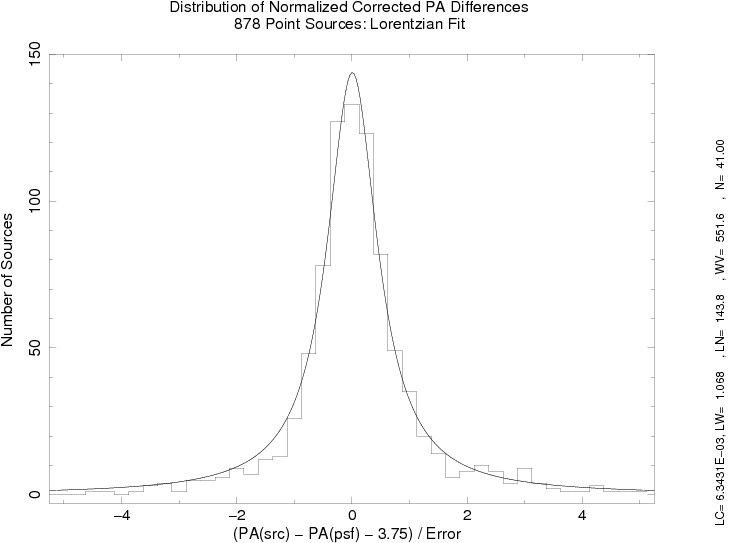## Position Angle Errors

In the Chandra Source Catalog, ellipses are fit to sources and PSFs to define the spatial extent of each. The parameters which describe these fitted ellipses in the CSC are the major and minor axis of each ellipse, the position angle of the major axis of the ellipse, and the errors on these quantities. The following describes how the position angle errors reported in the CSC are estimated, based on the errors and values of the ellipse major and minor axes.

### Position angle error expression

Since the error on position angle is related to the eccentricity of the ellipse, in particular the range of eccentricities allowed by the error bounds of the major and minor axes, the following expression was used to quantify the position angle error:

PA_ERR = atan2 ((MJR - MJR_ERR), (MNR + MNR_ERR)) - atan2 ((MJR + MJR_ERR), (MNR - MNR_ERR))

Here, 'MJR' and 'MNR' represent the ellipse major and minor axes, 'MJR_ERR' and 'MNR_ERR' their associated errors, and 'atan2' is assumed to have the parameter order atan2(x, y) (the arctangent of x/y given x and y).

Test

The position angle error expression was tested on the fitted ellipse parameters of 878 point sources and their PSFs. For sources classified as point sources, it is expected that the position angles of the raw fitted source and fitted PSF be the same. Therefore, the differences of those two position angles for all of the sources in the sample should provide a reliable error distribution.

For each source we derived PA_ERR(src), PA_ERR(psf), and PA(src)-PA(psf); we will refer to this last parameter as "PA". PA_ERR(src) and PA_ERR(psf) were added in quadrature to derive PA_ERR, and PA/PA_ERR yields a normalized difference PA_NORM.

Results

Fig. 1 presents PA plotted versus PA_ERR, and Fig. 2 PA_NORM versus PA_ERR. The latter should show a distribution that is independent of PA_ERR. The appearance of a systematic offset from zero of about 3.7 to 4 degrees will be the subject of a more thorough investigation at later time.

Fig. 3 presents the histogram distribution of the corrected PA_NORM, fitted with a Lorentzian function: center at 0.006 +/- 0.011, and gamma at 1.068 +/- 0.031. The variance in the fit is about 3.8. This represents a standard Cauchy distribution, i.e., the result is consistent with the notion that the expression offered above is a correct representation of the errors in the measured position angles.Figure 1. PA vs. PA_ERRFigure 2. PA_NORM vs. PA_ERRFigure 3. Histogram distribution of the corrected PA_NORM fitted with a Lorentzian function.